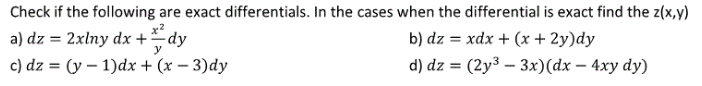Home / Answered Questions / Other / check-if-the-following-are-exact-differentials-in-the-cases-when-the-differential-is-exact-find-the--aw984

# (Solved): Check If The Following Are Exact Differentials. In The Cases When The Differential Is Exact Find The...Check if the following are exact differentials. In the cases when the differential is exact find the z(x,y) a) dz = 2xlny dx + dy b) dz = xdx + (x + 2y)dy c) dz = (y â€“ 1)dx + (x - 3)dy d) dz = (2y3 â€“ 3x)(dx - 4xy dy)

We have an Answer from Expert